## 原理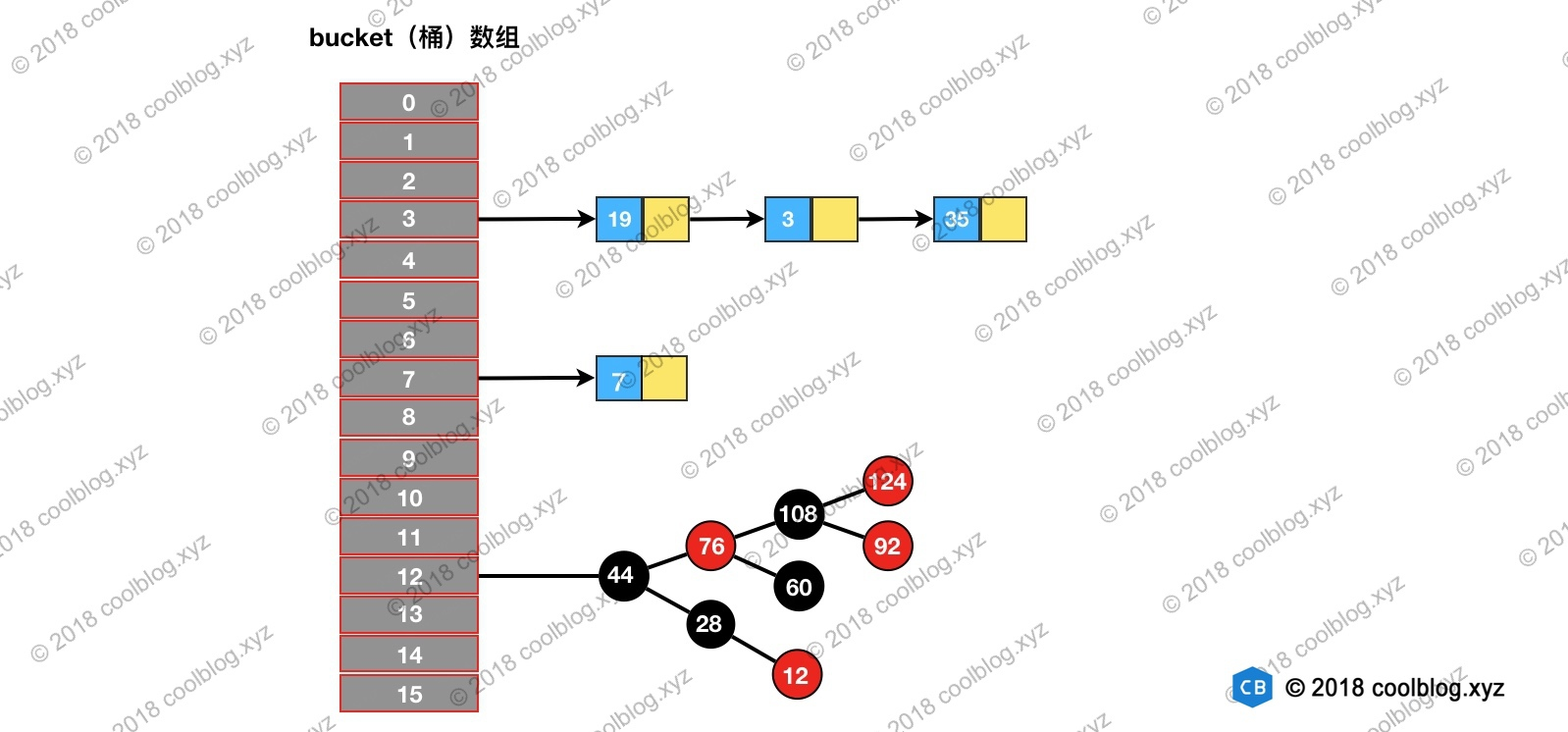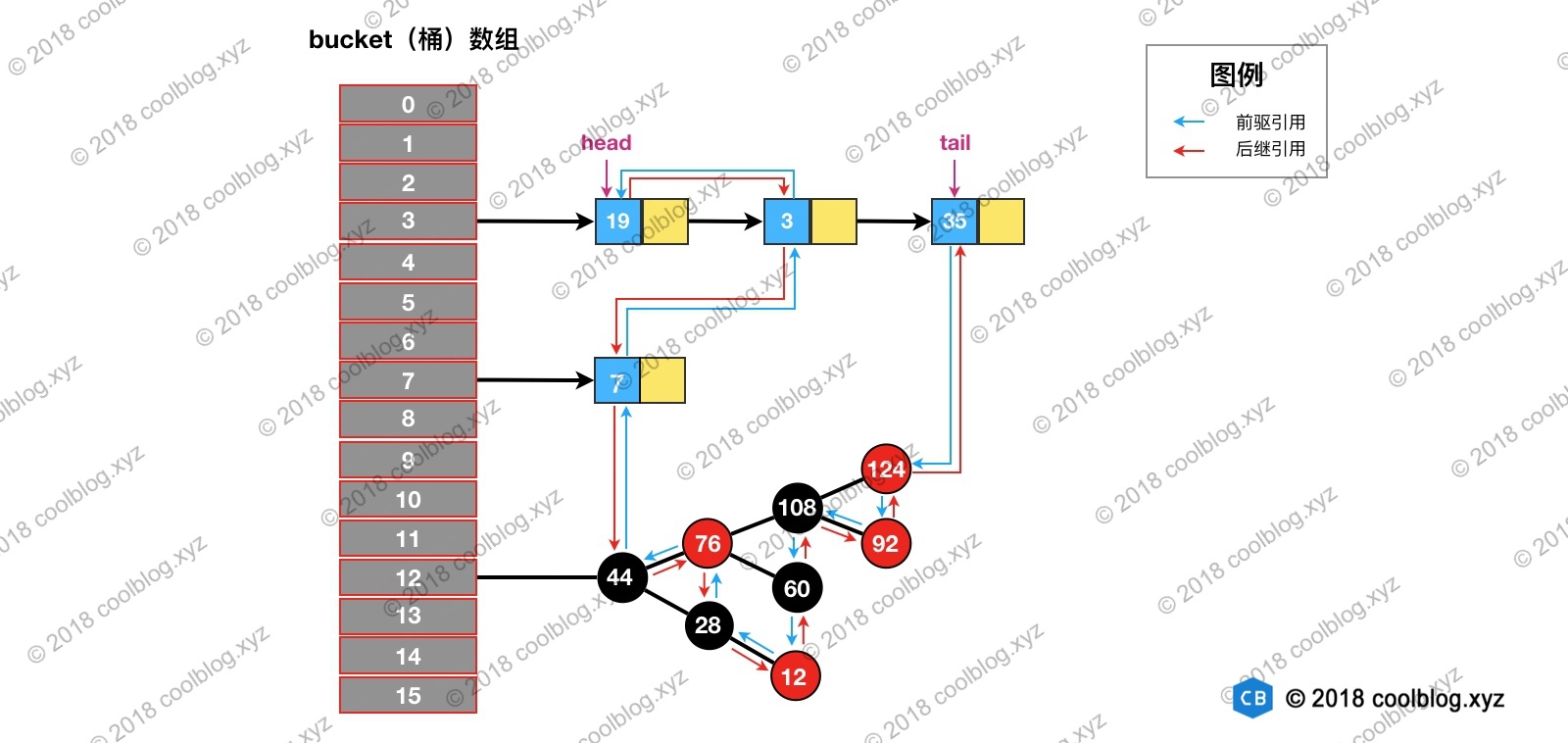## 源码分析

### Entry 的继承体系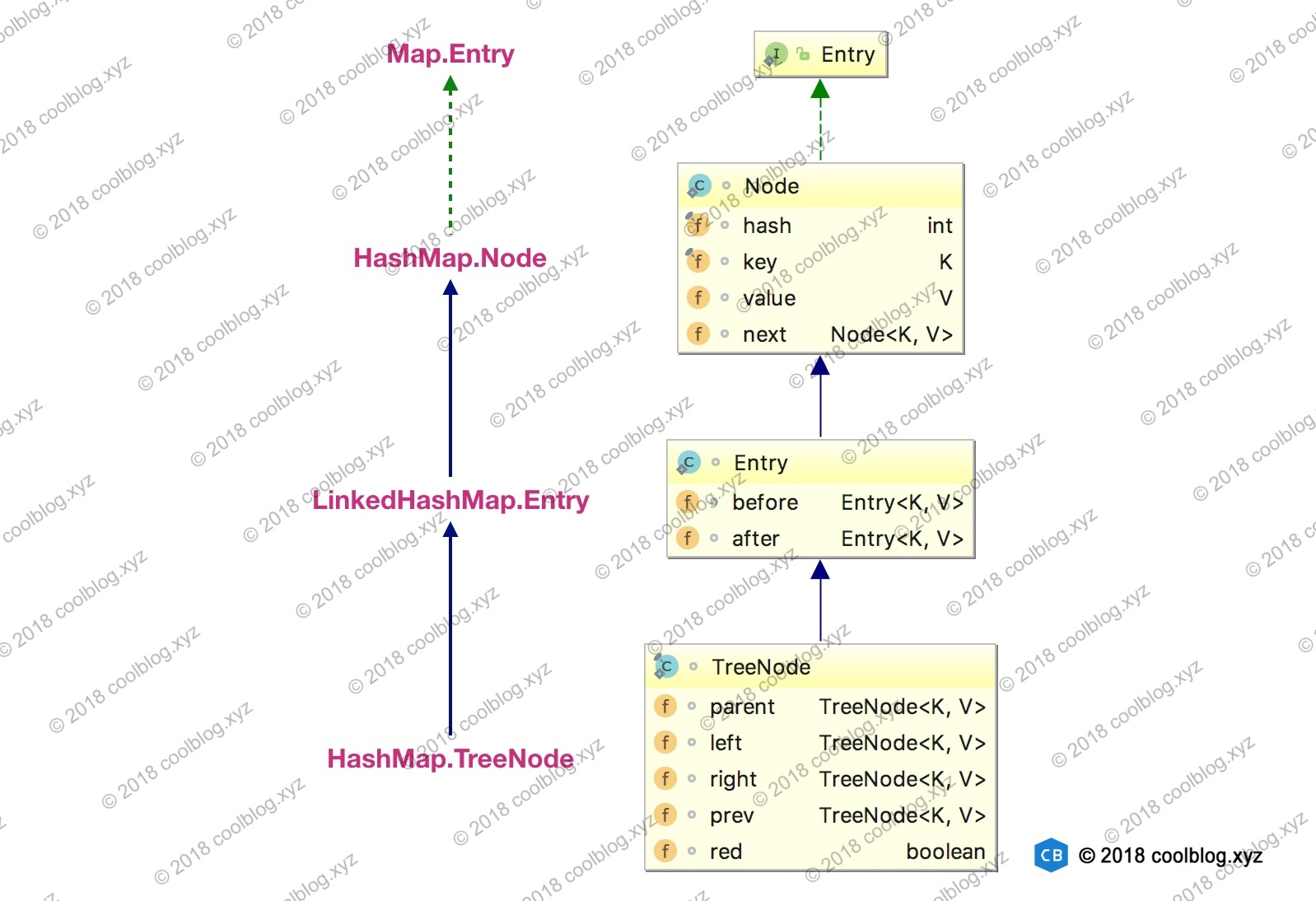Because TreeNodes are about twice the size of regular nodes, we
use them only when bins contain enough nodes to warrant use
(see TREEIFY_THRESHOLD). And when they become too small (due to
removal or resizing) they are converted back to plain bins. In
usages with well-distributed user hashCodes, tree bins are
rarely used.


### 链表的建立过程

// HashMap 中实现
public V put(K key, V value) {
return putVal(hash(key), key, value, false, true);
}

// HashMap 中实现
final V putVal(int hash, K key, V value, boolean onlyIfAbsent,
boolean evict) {
Node<K,V>[] tab; Node<K,V> p; int n, i;
if ((tab = table) == null || (n = tab.length) == 0) {...}
// 通过节点 hash 定位节点所在的桶位置，并检测桶中是否包含节点引用
if ((p = tab[i = (n - 1) & hash]) == null) {...}
else {
Node<K,V> e; K k;
if (p.hash == hash &&
((k = p.key) == key || (key != null && key.equals(k))))
e = p;
else if (p instanceof TreeNode) {...}
else {
// 遍历链表，并统计链表长度
for (int binCount = 0; ; ++binCount) {
// 未在单链表中找到要插入的节点，将新节点接在单链表的后面
if ((e = p.next) == null) {
p.next = newNode(hash, key, value, null);
if (binCount >= TREEIFY_THRESHOLD - 1) {...}
break;
}
// 插入的节点已经存在于单链表中
if (e.hash == hash &&
((k = e.key) == key || (key != null && key.equals(k))))
break;
p = e;
}
}
if (e != null) { // existing mapping for key
V oldValue = e.value;
if (!onlyIfAbsent || oldValue == null) {...}
afterNodeAccess(e);    // 回调方法，后续说明
return oldValue;
}
}
++modCount;
if (++size > threshold) {...}
afterNodeInsertion(evict);    // 回调方法，后续说明
return null;
}

// HashMap 中实现
Node<K,V> newNode(int hash, K key, V value, Node<K,V> next) {
return new Node<>(hash, key, value, next);
}

Node<K,V> newNode(int hash, K key, V value, Node<K,V> e) {
// 将 Entry 接在双向链表的尾部
return p;
}

tail = p;
// last 为 null，表明链表还未建立
if (last == null)
else {
// 将新节点 p 接在链表尾部
p.before = last;
last.after = p;
}
}// Callbacks to allow LinkedHashMap post-actions
void afterNodeAccess(Node<K,V> p) { }
void afterNodeInsertion(boolean evict) { }
void afterNodeRemoval(Node<K,V> p) { }


### 链表节点的删除过程

// HashMap 中实现
public V remove(Object key) {
Node<K,V> e;
return (e = removeNode(hash(key), key, null, false, true)) == null ?
null : e.value;
}

// HashMap 中实现
final Node<K,V> removeNode(int hash, Object key, Object value,
boolean matchValue, boolean movable) {
Node<K,V>[] tab; Node<K,V> p; int n, index;
if ((tab = table) != null && (n = tab.length) > 0 &&
(p = tab[index = (n - 1) & hash]) != null) {
Node<K,V> node = null, e; K k; V v;
if (p.hash == hash &&
((k = p.key) == key || (key != null && key.equals(k))))
node = p;
else if ((e = p.next) != null) {
if (p instanceof TreeNode) {...}
else {
// 遍历单链表，寻找要删除的节点，并赋值给 node 变量
do {
if (e.hash == hash &&
((k = e.key) == key ||
(key != null && key.equals(k)))) {
node = e;
break;
}
p = e;
} while ((e = e.next) != null);
}
}
if (node != null && (!matchValue || (v = node.value) == value ||
(value != null && value.equals(v)))) {
if (node instanceof TreeNode) {...}
// 将要删除的节点从单链表中移除
else if (node == p)
tab[index] = node.next;
else
p.next = node.next;
++modCount;
--size;
afterNodeRemoval(node);    // 调用删除回调方法进行后续操作
return node;
}
}
return null;
}

void afterNodeRemoval(Node<K,V> e) { // unlink
(LinkedHashMap.Entry<K,V>)e, b = p.before, a = p.after;
// 将 p 节点的前驱后后继引用置空
p.before = p.after = null;
// b 为 null，表明 p 是头节点
if (b == null)
else
b.after = a;
// a 为 null，表明 p 是尾节点
if (a == null)
tail = b;
else
a.before = b;
}


1. 根据 hash 定位到桶位置
2. 遍历链表或调用红黑树相关的删除方法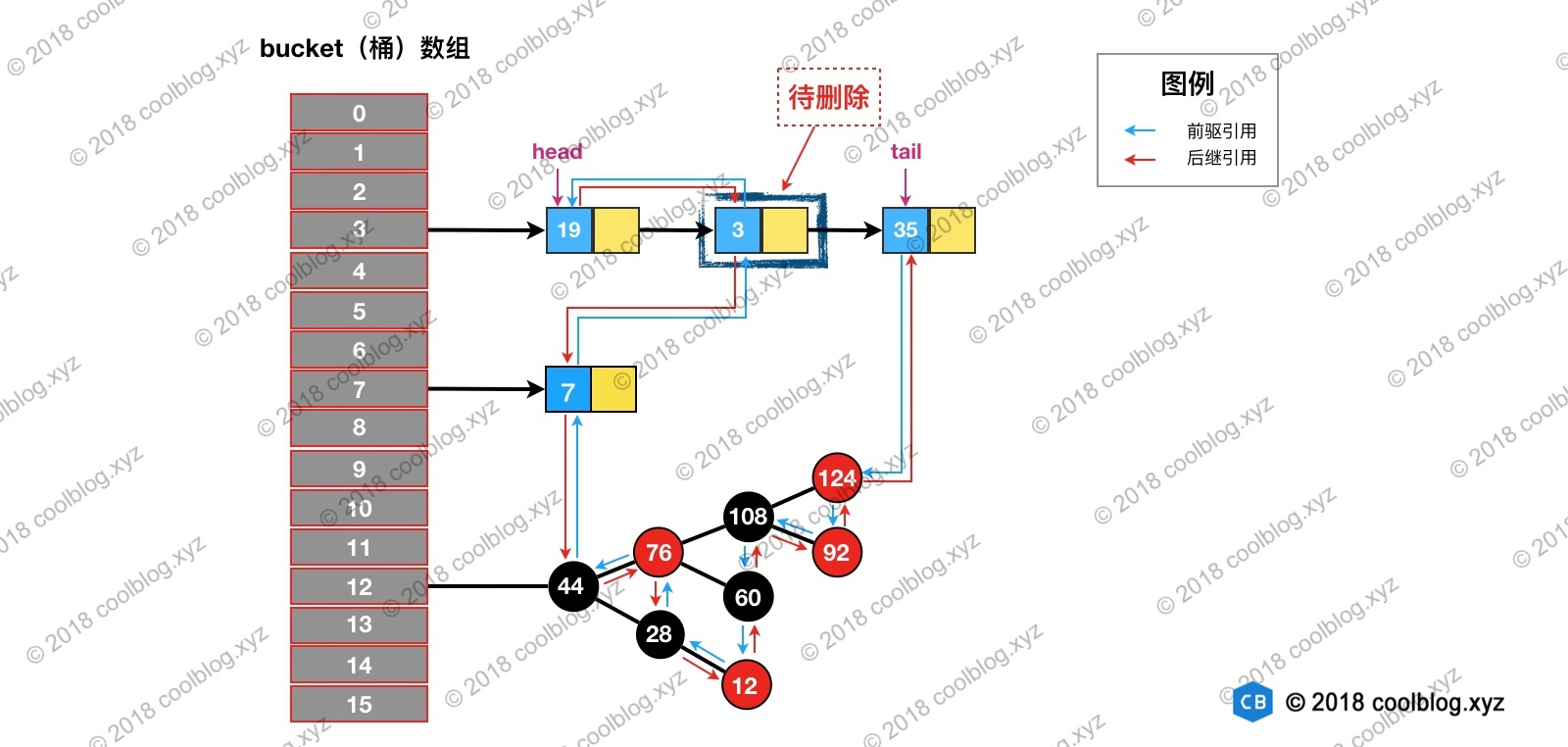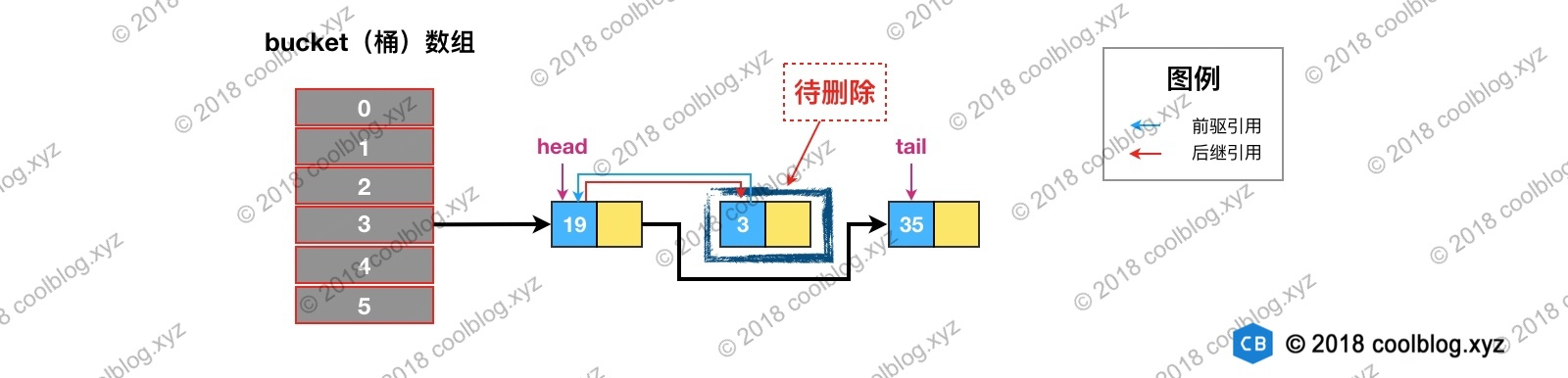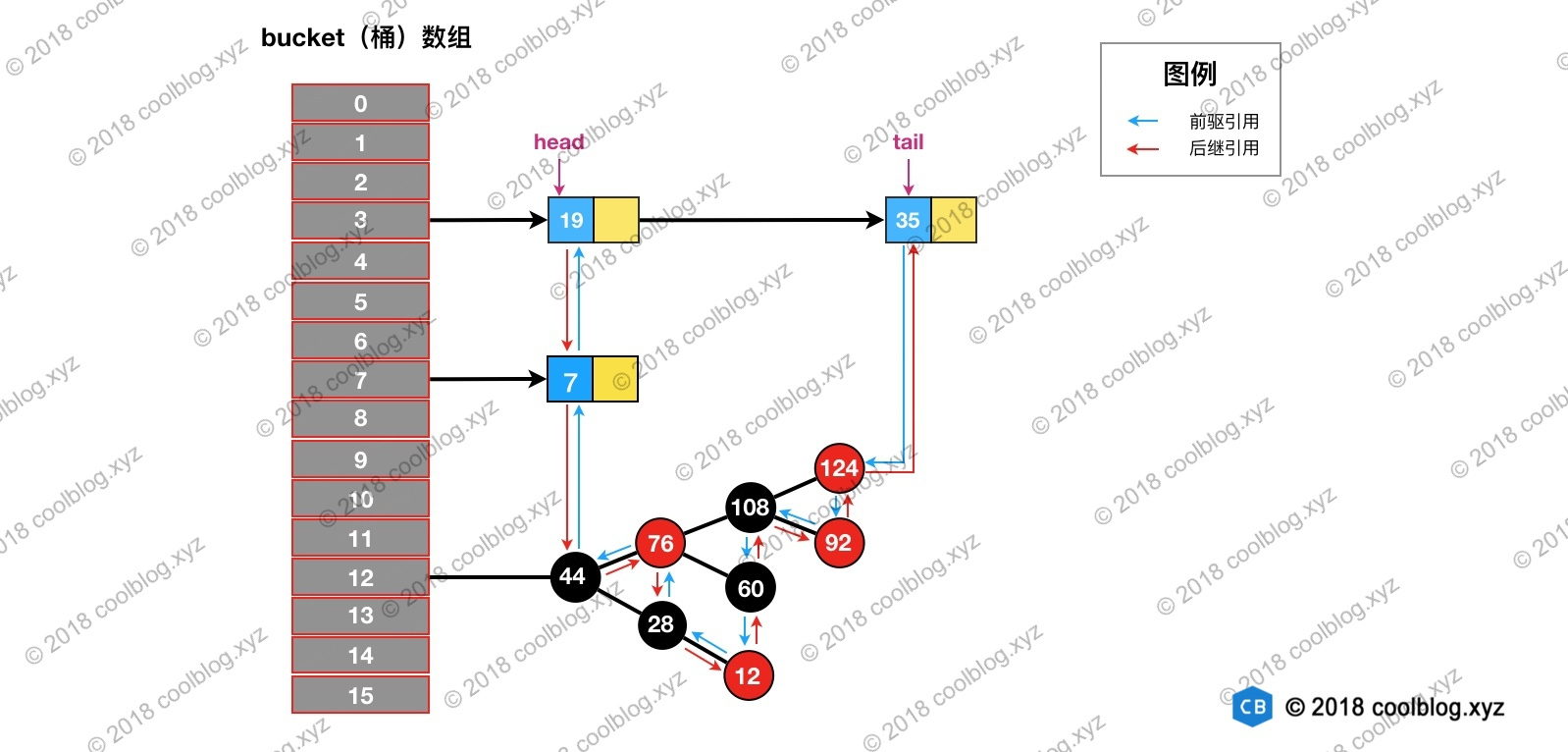### 访问顺序的维护过程

// LinkedHashMap 中覆写
public V get(Object key) {
Node<K,V> e;
if ((e = getNode(hash(key), key)) == null)
return null;
// 如果 accessOrder 为 true，则调用 afterNodeAccess 将被访问节点移动到链表最后
if (accessOrder)
afterNodeAccess(e);
return e.value;
}

void afterNodeAccess(Node<K,V> e) { // move node to last
if (accessOrder && (last = tail) != e) {
(LinkedHashMap.Entry<K,V>)e, b = p.before, a = p.after;
p.after = null;
// 如果 b 为 null，表明 p 为头节点
if (b == null)
else
b.after = a;

if (a != null)
a.before = b;
/*
* 这里存疑，父条件分支已经确保节点 e 不会是尾节点，
* 那么 e.after 必然不会为 null，不知道 else 分支有什么作用
*/
else
last = b;

if (last == null)
else {
// 将 p 接在链表的最后
p.before = last;
last.after = p;
}
tail = p;
++modCount;
}
}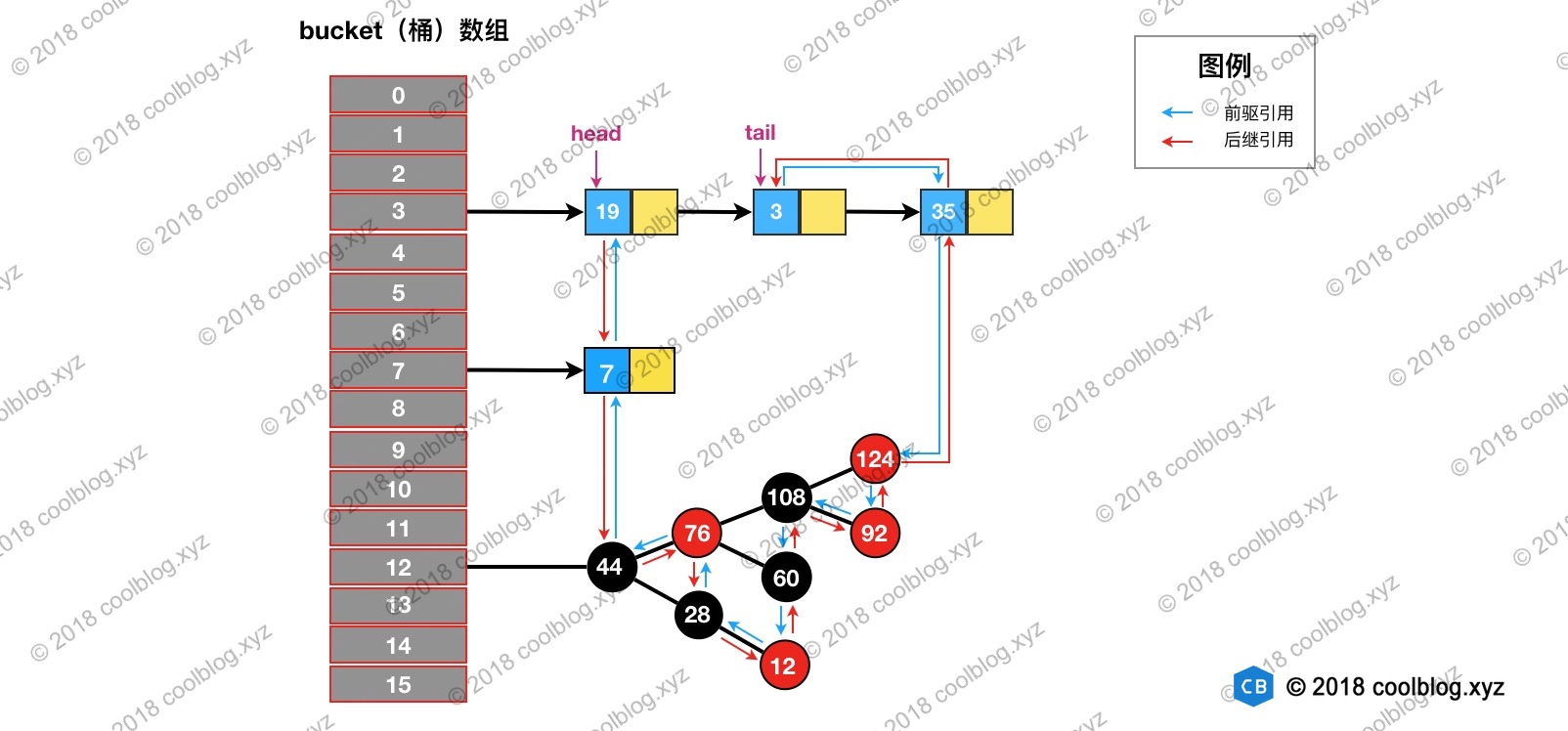void afterNodeInsertion(boolean evict) { // possibly remove eldest
// 根据条件判断是否移除最近最少被访问的节点
if (evict && (first = head) != null && removeEldestEntry(first)) {
K key = first.key;
removeNode(hash(key), key, null, false, true);
}
}

// 移除最近最少被访问条件之一，通过覆盖此方法可实现不同策略的缓存
protected boolean removeEldestEntry(Map.Entry<K,V> eldest) {
return false;
}


public class SimpleCache<K, V> extends LinkedHashMap<K, V> {

private static final int MAX_NODE_NUM = 100;

private int limit;

public SimpleCache() {
this(MAX_NODE_NUM);
}

public SimpleCache(int limit) {
super(limit, 0.75f, true);
this.limit = limit;
}

public V save(K key, V val) {
return put(key, val);
}

public V getOne(K key) {
return get(key);
}

public boolean exists(K key) {
return containsKey(key);
}

/**
* 判断节点数是否超限
* @param eldest
* @return 超限返回 true，否则返回 false
*/
@Override
protected boolean removeEldestEntry(Map.Entry<K, V> eldest) {
return size() > limit;
}
}


public class SimpleCacheTest {

@Test
public void test() throws Exception {
SimpleCache<Integer, Integer> cache = new SimpleCache<>(3);

for (int i = 0; i < 10; i++) {
cache.save(i, i * i);
}

System.out.println("插入10个键值对后，缓存内容：");
System.out.println(cache + "\n");

System.out.println("访问键值为7的节点后，缓存内容：");
cache.getOne(7);
System.out.println(cache + "\n");

System.out.println("插入键值为1的键值对后，缓存内容：");
cache.save(1, 1);
System.out.println(cache);
}
}


插入10个键值对后，缓存内容：
{7=49, 8=64, 9=81}

{8=64, 9=81, 7=49}

{9=81, 7=49, 1=1}


## 总结

11-2704-02
03-1510万+
04-114209
04-171217
03-22186
09-15152
10-18149
12-1029
07-01355